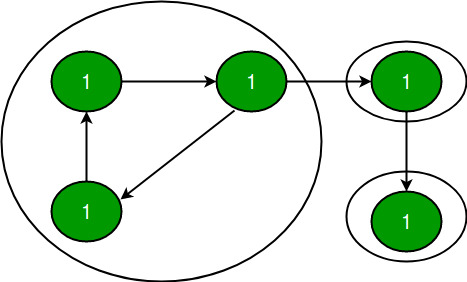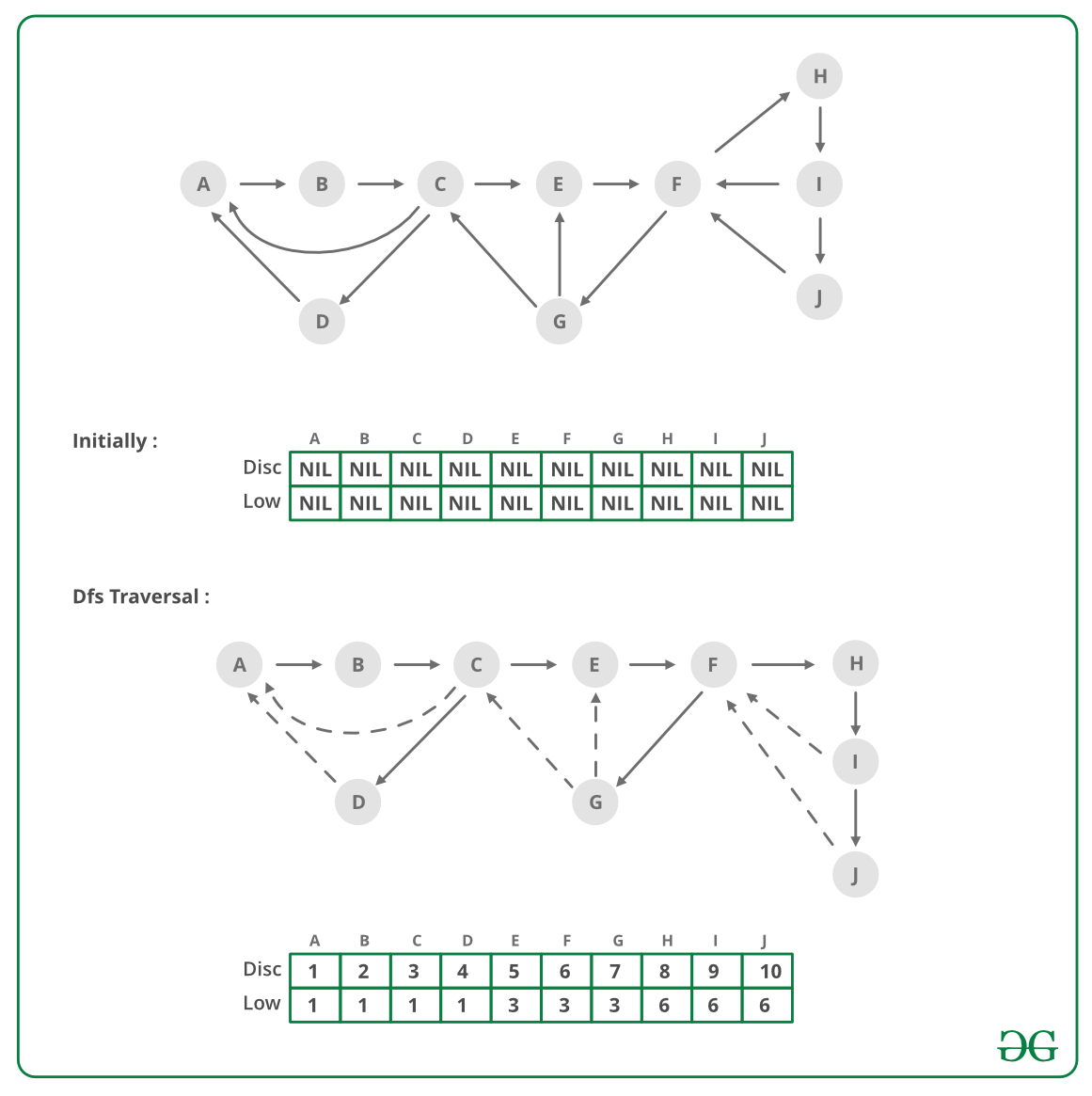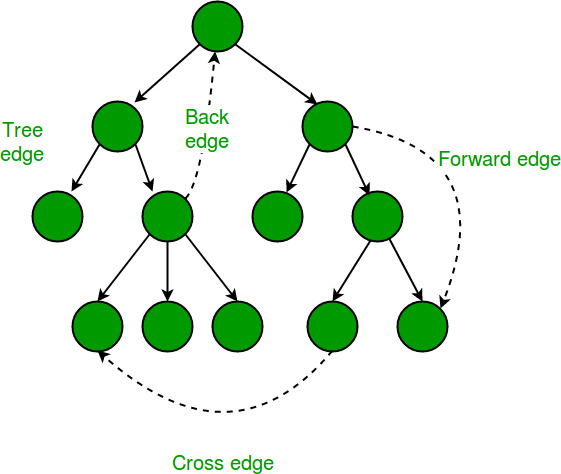GeeksforGeeks App
Open AppBrowser
Continue

# Tarjan’s Algorithm to find Strongly Connected Components

A directed graph is strongly connected if there is a path between all pairs of vertices. A strongly connected component (SCC) of a directed graph is a maximal strongly connected subgraph. For example, there are 3 SCCs in the following graph:We have discussed Kosaraju’s algorithm for strongly connected components. The previously discussed algorithm requires two DFS traversals of a Graph. In this post, Tarjan’s algorithm is discussed that requires only one DFS traversal:

Tarjan Algorithm is based on the following facts:

• DFS search produces a DFS tree/forest
• Strongly Connected Components form subtrees of the DFS tree.
• If we can find the head of such subtrees, we can print/store all the nodes in that subtree (including the head) and that will be one SCC.
• There is no back edge from one SCC to another (There can be cross edges, but cross edges will not be used while processing the graph).

To find the head of an SCC, we calculate the disc and low array (as done for articulation point, bridge, and biconnected component). As discussed in the previous posts, low[u] indicates the earliest visited vertex (the vertex with minimum discovery time) that can be reached from a subtree rooted with u. A node u is head if disc[u] = low[u]

Below is an illustration of the above approach:To solve the problem follow the below idea:

Strongly Connected Component relates to directed graph only, but Disc and Low values relate to both directed and undirected graph, so in the above pic we have taken an undirected graph.

In the above Figure, we have shown a graph and one of the DFS trees (There could be different DFS trees on the same graph depending on the order in which edges are traversed). In a DFS tree, continuous arrows are tree edges, and dashed arrows are back edges (DFS Tree Edges ). Disc and Low values are shown in the Figure for every node as (Disc/Low).

Disc: This is the time when a node is visited 1st time while DFS traversal. For nodes A, B, C, .., and J in the DFS tree, Disc values are 1, 2, 3, .., 10.

Low: In the DFS tree, Tree edges take us forward, from the ancestor node to one of its descendants. For example, from node C, tree edges can take us to node G, node I, etc. Back edges take us backward, from a descendant node to one of its ancestors.

For example: From node G, the Back edges take us to E or C. If we look at both the Tree and Back edges together, then we can see that if we start traversal from one node, we may go down the tree via Tree edges and then go up via back edges.

For example, from node E, we can go down to G and then go up to C. Similarly from E, we can go down to I or J and then go up to F. “Low” value of a node tells the topmost reachable ancestor (with minimum possible Disc value) via the subtree of that node. So for any node, a Low value is equal to its Disc value anyway (A node is the ancestor of itself). Then we look into its subtree and see if there is any node that can take us to any of its ancestors.

If there are multiple back edges in the subtree that take us to different ancestors, then we take the one with the minimum Disc value (i.e. the topmost one). If we look at node F, it has two subtrees. Subtree with node G takes us to E and C. The other subtree takes us back to F only. Here topmost ancestor is C where F can reach and so the Low value of F is 3 (The Disc value of C).

Based on the above discussion, it should be clear that the Low values of B, C, and D are 1 (As A is the topmost node where B, C, and D can reach). In the same way, the Low values of E, F, and G are 3, and the Low values of H, I, and J are 6.
For any node u, when DFS starts, Low will be set to its Disc 1st

Then later on DFS will be performed on each of its children v one by one, Low value of u can change in two cases:

• Case1 (Tree Edge): If node v is not visited already, then after the DFS of v is complete, a minimum of low[u] and low[v] will be updated to low[u].
low[u] = min(low[u], low[v]);
• Case 2 (Back Edge): When child v is already visited, then a minimum of low[u] and Disc[v] will be updated to low[u].
low[u] = min(low[u], disc[v]);

In case two, can we take low[v] instead of the disc[v] ?? The answer is NO. If you can think why the answer is NO, you probably understood the Low and Disc concept.Same Low and Disc values help to solve other graph problems like articulation point, bridge, and biconnected component. To track the subtree rooted at the head, we can use a stack (keep pushing the node while visiting). When a head node is found, pop all nodes from the stack till you get the head out of the stack. To make sure, we don’t consider cross edges, when we reach a node that is already visited, we should process the visited node only if it is present in the stack, or else ignore the node.

Below is the implementation of Tarjan’s algorithm to print all SCCs.

## C++

 `// A C++ program to find strongly connected components in a``// given directed graph using Tarjan's algorithm (single``// DFS)``#include ``#define NIL -1``using` `namespace` `std;` `// A class that represents an directed graph``class` `Graph {``    ``int` `V; ``// No. of vertices``    ``list<``int``>* adj; ``// A dynamic array of adjacency lists` `    ``// A Recursive DFS based function used by SCC()``    ``void` `SCCUtil(``int` `u, ``int` `disc[], ``int` `low[],``                 ``stack<``int``>* st, ``bool` `stackMember[]);` `public``:``    ``Graph(``int` `V); ``// Constructor``    ``void` `addEdge(``int` `v,``                 ``int` `w); ``// function to add an edge to graph``    ``void` `SCC(); ``// prints strongly connected components``};` `Graph::Graph(``int` `V)``{``    ``this``->V = V;``    ``adj = ``new` `list<``int``>[V];``}` `void` `Graph::addEdge(``int` `v, ``int` `w) { adj[v].push_back(w); }` `// A recursive function that finds and prints strongly``// connected components using DFS traversal u --> The vertex``// to be visited next disc[] --> Stores discovery times of``// visited vertices low[] -- >> earliest visited vertex (the``// vertex with minimum``//             discovery time) that can be reached from``//             subtree rooted with current vertex``// *st -- >> To store all the connected ancestors (could be``// part``//         of SCC)``// stackMember[] --> bit/index array for faster check``// whether``//                 a node is in stack``void` `Graph::SCCUtil(``int` `u, ``int` `disc[], ``int` `low[],``                    ``stack<``int``>* st, ``bool` `stackMember[])``{``    ``// A static variable is used for simplicity, we can``    ``// avoid use of static variable by passing a pointer.``    ``static` `int` `time` `= 0;` `    ``// Initialize discovery time and low value``    ``disc[u] = low[u] = ++``time``;``    ``st->push(u);``    ``stackMember[u] = ``true``;` `    ``// Go through all vertices adjacent to this``    ``list<``int``>::iterator i;``    ``for` `(i = adj[u].begin(); i != adj[u].end(); ++i) {``        ``int` `v = *i; ``// v is current adjacent of 'u'` `        ``// If v is not visited yet, then recur for it``        ``if` `(disc[v] == -1) {``            ``SCCUtil(v, disc, low, st, stackMember);` `            ``// Check if the subtree rooted with 'v' has a``            ``// connection to one of the ancestors of 'u'``            ``// Case 1 (per above discussion on Disc and Low``            ``// value)``            ``low[u] = min(low[u], low[v]);``        ``}` `        ``// Update low value of 'u' only of 'v' is still in``        ``// stack (i.e. it's a back edge, not cross edge).``        ``// Case 2 (per above discussion on Disc and Low``        ``// value)``        ``else` `if` `(stackMember[v] == ``true``)``            ``low[u] = min(low[u], disc[v]);``    ``}` `    ``// head node found, pop the stack and print an SCC``    ``int` `w = 0; ``// To store stack extracted vertices``    ``if` `(low[u] == disc[u]) {``        ``while` `(st->top() != u) {``            ``w = (``int``)st->top();``            ``cout << w << ``" "``;``            ``stackMember[w] = ``false``;``            ``st->pop();``        ``}``        ``w = (``int``)st->top();``        ``cout << w << ``"\n"``;``        ``stackMember[w] = ``false``;``        ``st->pop();``    ``}``}` `// The function to do DFS traversal. It uses SCCUtil()``void` `Graph::SCC()``{``    ``int``* disc = ``new` `int``[V];``    ``int``* low = ``new` `int``[V];``    ``bool``* stackMember = ``new` `bool``[V];``    ``stack<``int``>* st = ``new` `stack<``int``>();` `    ``// Initialize disc and low, and stackMember arrays``    ``for` `(``int` `i = 0; i < V; i++) {``        ``disc[i] = NIL;``        ``low[i] = NIL;``        ``stackMember[i] = ``false``;``    ``}` `    ``// Call the recursive helper function to find strongly``    ``// connected components in DFS tree with vertex 'i'``    ``for` `(``int` `i = 0; i < V; i++)``        ``if` `(disc[i] == NIL)``            ``SCCUtil(i, disc, low, st, stackMember);``}` `// Driver program to test above function``int` `main()``{``    ``cout << ``"\nSCCs in first graph \n"``;``    ``Graph g1(5);``    ``g1.addEdge(1, 0);``    ``g1.addEdge(0, 2);``    ``g1.addEdge(2, 1);``    ``g1.addEdge(0, 3);``    ``g1.addEdge(3, 4);``    ``g1.SCC();` `    ``cout << ``"\nSCCs in second graph \n"``;``    ``Graph g2(4);``    ``g2.addEdge(0, 1);``    ``g2.addEdge(1, 2);``    ``g2.addEdge(2, 3);``    ``g2.SCC();` `    ``cout << ``"\nSCCs in third graph \n"``;``    ``Graph g3(7);``    ``g3.addEdge(0, 1);``    ``g3.addEdge(1, 2);``    ``g3.addEdge(2, 0);``    ``g3.addEdge(1, 3);``    ``g3.addEdge(1, 4);``    ``g3.addEdge(1, 6);``    ``g3.addEdge(3, 5);``    ``g3.addEdge(4, 5);``    ``g3.SCC();` `    ``cout << ``"\nSCCs in fourth graph \n"``;``    ``Graph g4(11);``    ``g4.addEdge(0, 1);``    ``g4.addEdge(0, 3);``    ``g4.addEdge(1, 2);``    ``g4.addEdge(1, 4);``    ``g4.addEdge(2, 0);``    ``g4.addEdge(2, 6);``    ``g4.addEdge(3, 2);``    ``g4.addEdge(4, 5);``    ``g4.addEdge(4, 6);``    ``g4.addEdge(5, 6);``    ``g4.addEdge(5, 7);``    ``g4.addEdge(5, 8);``    ``g4.addEdge(5, 9);``    ``g4.addEdge(6, 4);``    ``g4.addEdge(7, 9);``    ``g4.addEdge(8, 9);``    ``g4.addEdge(9, 8);``    ``g4.SCC();` `    ``cout << ``"\nSCCs in fifth graph \n"``;``    ``Graph g5(5);``    ``g5.addEdge(0, 1);``    ``g5.addEdge(1, 2);``    ``g5.addEdge(2, 3);``    ``g5.addEdge(2, 4);``    ``g5.addEdge(3, 0);``    ``g5.addEdge(4, 2);``    ``g5.SCC();` `    ``return` `0;``}`

## Java

 `// Java program to find strongly connected``// components in a given directed graph``// using Tarjan's algorithm (single DFS)``import` `java.io.*;``import` `java.util.*;` `// This class represents a directed graph``// using adjacency list representation``class` `Graph {` `    ``// No. of vertices``    ``private` `int` `V;` `    ``// Adjacency Lists``    ``private` `LinkedList adj[];``    ``private` `int` `Time;` `    ``// Constructor``    ``@SuppressWarnings``(``"unchecked"``) Graph(``int` `v)``    ``{``        ``V = v;``        ``adj = ``new` `LinkedList[v];` `        ``for` `(``int` `i = ``0``; i < v; ++i)``            ``adj[i] = ``new` `LinkedList();` `        ``Time = ``0``;``    ``}` `    ``// Function to add an edge into the graph``    ``void` `addEdge(``int` `v, ``int` `w) { adj[v].add(w); }` `    ``// A recursive function that finds and prints strongly``    ``// connected components using DFS traversal``    ``// u --> The vertex to be visited next``    ``// disc[] --> Stores discovery times of visited vertices``    ``// low[] -- >> earliest visited vertex (the vertex with``    ``//             minimum discovery time) that can be``    ``//             reached from subtree rooted with current``    ``//             vertex``    ``// st -- >> To store all the connected ancestors (could``    ``// be part``    ``//         of SCC)``    ``// stackMember[] --> bit/index array for faster check``    ``//                   whether a node is in stack``    ``void` `SCCUtil(``int` `u, ``int` `low[], ``int` `disc[],``                 ``boolean` `stackMember[], Stack st)``    ``{` `        ``// Initialize discovery time and low value``        ``disc[u] = Time;``        ``low[u] = Time;``        ``Time += ``1``;``        ``stackMember[u] = ``true``;``        ``st.push(u);` `        ``int` `n;` `        ``// Go through all vertices adjacent to this``        ``Iterator i = adj[u].iterator();` `        ``while` `(i.hasNext()) {``            ``n = i.next();` `            ``if` `(disc[n] == -``1``) {``                ``SCCUtil(n, low, disc, stackMember, st);` `                ``// Check if the subtree rooted with v``                ``// has a connection to one of the``                ``// ancestors of u``                ``// Case 1 (per above discussion on``                ``// Disc and Low value)``                ``low[u] = Math.min(low[u], low[n]);``            ``}``            ``else` `if` `(stackMember[n] == ``true``) {` `                ``// Update low value of 'u' only if 'v' is``                ``// still in stack (i.e. it's a back edge,``                ``// not cross edge).``                ``// Case 2 (per above discussion on Disc``                ``// and Low value)``                ``low[u] = Math.min(low[u], disc[n]);``            ``}``        ``}` `        ``// head node found, pop the stack and print an SCC``        ``// To store stack extracted vertices``        ``int` `w = -``1``;``        ``if` `(low[u] == disc[u]) {``            ``while` `(w != u) {``                ``w = (``int``)st.pop();``                ``System.out.print(w + ``" "``);``                ``stackMember[w] = ``false``;``            ``}``            ``System.out.println();``        ``}``    ``}` `    ``// The function to do DFS traversal.``    ``// It uses SCCUtil()``    ``void` `SCC()``    ``{` `        ``// Mark all the vertices as not visited``        ``// and Initialize parent and visited,``        ``// and ap(articulation point) arrays``        ``int` `disc[] = ``new` `int``[V];``        ``int` `low[] = ``new` `int``[V];``        ``for` `(``int` `i = ``0``; i < V; i++) {``            ``disc[i] = -``1``;``            ``low[i] = -``1``;``        ``}` `        ``boolean` `stackMember[] = ``new` `boolean``[V];``        ``Stack st = ``new` `Stack();` `        ``// Call the recursive helper function``        ``// to find articulation points``        ``// in DFS tree rooted with vertex 'i'``        ``for` `(``int` `i = ``0``; i < V; i++) {``            ``if` `(disc[i] == -``1``)``                ``SCCUtil(i, low, disc, stackMember, st);``        ``}``    ``}` `    ``// Driver code``    ``public` `static` `void` `main(String args[])``    ``{` `        ``// Create a graph given in the above diagram``        ``Graph g1 = ``new` `Graph(``5``);` `        ``g1.addEdge(``1``, ``0``);``        ``g1.addEdge(``0``, ``2``);``        ``g1.addEdge(``2``, ``1``);``        ``g1.addEdge(``0``, ``3``);``        ``g1.addEdge(``3``, ``4``);``        ``System.out.println(``"SSC in first graph "``);``        ``g1.SCC();` `        ``Graph g2 = ``new` `Graph(``4``);``        ``g2.addEdge(``0``, ``1``);``        ``g2.addEdge(``1``, ``2``);``        ``g2.addEdge(``2``, ``3``);``        ``System.out.println(``"\nSSC in second graph "``);``        ``g2.SCC();` `        ``Graph g3 = ``new` `Graph(``7``);``        ``g3.addEdge(``0``, ``1``);``        ``g3.addEdge(``1``, ``2``);``        ``g3.addEdge(``2``, ``0``);``        ``g3.addEdge(``1``, ``3``);``        ``g3.addEdge(``1``, ``4``);``        ``g3.addEdge(``1``, ``6``);``        ``g3.addEdge(``3``, ``5``);``        ``g3.addEdge(``4``, ``5``);``        ``System.out.println(``"\nSSC in third graph "``);``        ``g3.SCC();` `        ``Graph g4 = ``new` `Graph(``11``);``        ``g4.addEdge(``0``, ``1``);``        ``g4.addEdge(``0``, ``3``);``        ``g4.addEdge(``1``, ``2``);``        ``g4.addEdge(``1``, ``4``);``        ``g4.addEdge(``2``, ``0``);``        ``g4.addEdge(``2``, ``6``);``        ``g4.addEdge(``3``, ``2``);``        ``g4.addEdge(``4``, ``5``);``        ``g4.addEdge(``4``, ``6``);``        ``g4.addEdge(``5``, ``6``);``        ``g4.addEdge(``5``, ``7``);``        ``g4.addEdge(``5``, ``8``);``        ``g4.addEdge(``5``, ``9``);``        ``g4.addEdge(``6``, ``4``);``        ``g4.addEdge(``7``, ``9``);``        ``g4.addEdge(``8``, ``9``);``        ``g4.addEdge(``9``, ``8``);``        ``System.out.println(``"\nSSC in fourth graph "``);``        ``g4.SCC();` `        ``Graph g5 = ``new` `Graph(``5``);``        ``g5.addEdge(``0``, ``1``);``        ``g5.addEdge(``1``, ``2``);``        ``g5.addEdge(``2``, ``3``);``        ``g5.addEdge(``2``, ``4``);``        ``g5.addEdge(``3``, ``0``);``        ``g5.addEdge(``4``, ``2``);``        ``System.out.println(``"\nSSC in fifth graph "``);``        ``g5.SCC();``    ``}``}` `// This code is contributed by``// Prateek Gupta (@prateekgupta10)`

## Python3

 `# Python program to find strongly connected components in a given``# directed graph using Tarjan's algorithm (single DFS)``#Complexity : O(V+E)` `from` `collections ``import` `defaultdict` `# This class represents an directed graph``# using adjacency list representation`  `class` `Graph:` `    ``def` `__init__(``self``, vertices):``        ``# No. of vertices``        ``self``.V ``=` `vertices` `        ``# default dictionary to store graph``        ``self``.graph ``=` `defaultdict(``list``)` `        ``self``.Time ``=` `0` `    ``# function to add an edge to graph``    ``def` `addEdge(``self``, u, v):``        ``self``.graph[u].append(v)` `    ``'''A recursive function that find finds and prints strongly connected``    ``components using DFS traversal``    ``u --> The vertex to be visited next``    ``disc[] --> Stores discovery times of visited vertices``    ``low[] -- >> earliest visited vertex (the vertex with minimum``                ``discovery time) that can be reached from subtree``                ``rooted with current vertex``     ``st -- >> To store all the connected ancestors (could be part``           ``of SCC)``     ``stackMember[] --> bit/index array for faster check whether``                  ``a node is in stack``    ``'''` `    ``def` `SCCUtil(``self``, u, low, disc, stackMember, st):` `        ``# Initialize discovery time and low value``        ``disc[u] ``=` `self``.Time``        ``low[u] ``=` `self``.Time``        ``self``.Time ``+``=` `1``        ``stackMember[u] ``=` `True``        ``st.append(u)` `        ``# Go through all vertices adjacent to this``        ``for` `v ``in` `self``.graph[u]:` `            ``# If v is not visited yet, then recur for it``            ``if` `disc[v] ``=``=` `-``1``:` `                ``self``.SCCUtil(v, low, disc, stackMember, st)` `                ``# Check if the subtree rooted with v has a connection to``                ``# one of the ancestors of u``                ``# Case 1 (per above discussion on Disc and Low value)``                ``low[u] ``=` `min``(low[u], low[v])` `            ``elif` `stackMember[v] ``=``=` `True``:` `                ``'''Update low value of 'u' only if 'v' is still in stack``                ``(i.e. it's a back edge, not cross edge).``                ``Case 2 (per above discussion on Disc and Low value) '''``                ``low[u] ``=` `min``(low[u], disc[v])` `        ``# head node found, pop the stack and print an SCC``        ``w ``=` `-``1`  `# To store stack extracted vertices``        ``if` `low[u] ``=``=` `disc[u]:``            ``while` `w !``=` `u:``                ``w ``=` `st.pop()``                ``print``(w, end``=``" "``)``                ``stackMember[w] ``=` `False` `            ``print``()` `    ``# The function to do DFS traversal.``    ``# It uses recursive SCCUtil()` `    ``def` `SCC(``self``):` `        ``# Mark all the vertices as not visited``        ``# and Initialize parent and visited,``        ``# and ap(articulation point) arrays``        ``disc ``=` `[``-``1``] ``*` `(``self``.V)``        ``low ``=` `[``-``1``] ``*` `(``self``.V)``        ``stackMember ``=` `[``False``] ``*` `(``self``.V)``        ``st ``=` `[]` `        ``# Call the recursive helper function``        ``# to find articulation points``        ``# in DFS tree rooted with vertex 'i'``        ``for` `i ``in` `range``(``self``.V):``            ``if` `disc[i] ``=``=` `-``1``:``                ``self``.SCCUtil(i, low, disc, stackMember, st)`  `# Create a graph given in the above diagram``g1 ``=` `Graph(``5``)``g1.addEdge(``1``, ``0``)``g1.addEdge(``0``, ``2``)``g1.addEdge(``2``, ``1``)``g1.addEdge(``0``, ``3``)``g1.addEdge(``3``, ``4``)``print``(``"SSC in first graph "``)``g1.SCC()` `g2 ``=` `Graph(``4``)``g2.addEdge(``0``, ``1``)``g2.addEdge(``1``, ``2``)``g2.addEdge(``2``, ``3``)``print``(``"\nSSC in second graph "``)``g2.SCC()`  `g3 ``=` `Graph(``7``)``g3.addEdge(``0``, ``1``)``g3.addEdge(``1``, ``2``)``g3.addEdge(``2``, ``0``)``g3.addEdge(``1``, ``3``)``g3.addEdge(``1``, ``4``)``g3.addEdge(``1``, ``6``)``g3.addEdge(``3``, ``5``)``g3.addEdge(``4``, ``5``)``print``(``"\nSSC in third graph "``)``g3.SCC()` `g4 ``=` `Graph(``11``)``g4.addEdge(``0``, ``1``)``g4.addEdge(``0``, ``3``)``g4.addEdge(``1``, ``2``)``g4.addEdge(``1``, ``4``)``g4.addEdge(``2``, ``0``)``g4.addEdge(``2``, ``6``)``g4.addEdge(``3``, ``2``)``g4.addEdge(``4``, ``5``)``g4.addEdge(``4``, ``6``)``g4.addEdge(``5``, ``6``)``g4.addEdge(``5``, ``7``)``g4.addEdge(``5``, ``8``)``g4.addEdge(``5``, ``9``)``g4.addEdge(``6``, ``4``)``g4.addEdge(``7``, ``9``)``g4.addEdge(``8``, ``9``)``g4.addEdge(``9``, ``8``)``print``(``"\nSSC in fourth graph "``)``g4.SCC()`  `g5 ``=` `Graph(``5``)``g5.addEdge(``0``, ``1``)``g5.addEdge(``1``, ``2``)``g5.addEdge(``2``, ``3``)``g5.addEdge(``2``, ``4``)``g5.addEdge(``3``, ``0``)``g5.addEdge(``4``, ``2``)``print``(``"\nSSC in fifth graph "``)``g5.SCC()` `# This code is contributed by Neelam Yadav`

## Javascript

 ``

## C#

 `using` `System;``using` `System.Collections.Generic;` `// This class represents a directed graph``// using adjacency list representation``class` `Graph``{``    ``// No. of vertices``    ``private` `int` `V;` `    ``// Adjacency Lists``    ``private` `List<``int``>[] adj;``    ``private` `int` `Time;` `    ``// Constructor``    ``public` `Graph(``int` `v)``    ``{``        ``V = v;``        ``adj = ``new` `List<``int``>[v];` `        ``for` `(``int` `i = 0; i < v; ++i)``            ``adj[i] = ``new` `List<``int``>();` `        ``Time = 0;``    ``}` `    ``// Function to add an edge into the graph``    ``public` `void` `addEdge(``int` `v, ``int` `w) { adj[v].Add(w); }` `    ``// A recursive function that finds and prints strongly``    ``// connected components using DFS traversal``    ``// u --> The vertex to be visited next``    ``// disc[] --> Stores discovery times of visited vertices``    ``// low[] -- >> earliest visited vertex (the vertex with``    ``//             minimum discovery time) that can be``    ``//             reached from subtree rooted with current``    ``//             vertex``    ``// st -- >> To store all the connected ancestors (could``    ``// be part``    ``//         of SCC)``    ``// stackMember[] --> bit/index array for faster check``    ``//                   whether a node is in stack``    ``private` `void` `SCCUtil(``int` `u, ``int``[] low, ``int``[] disc,``                 ``bool``[] stackMember, Stack<``int``> st)``    ``{` `        ``// Initialize discovery time and low value``        ``disc[u] = Time;``        ``low[u] = Time;``        ``Time += 1;``        ``stackMember[u] = ``true``;``        ``st.Push(u);` `        ``int` `n;` `        ``// Go through all vertices adjacent to this``        ``foreach` `(``int` `i ``in` `adj[u])``        ``{``            ``n = i;` `            ``if` `(disc[n] == -1)``            ``{``                ``SCCUtil(n, low, disc, stackMember, st);` `                ``// Check if the subtree rooted with v``                ``// has a connection to one of the``                ``// ancestors of u``                ``// Case 1 (per above discussion on``                ``// Disc and Low value)``                ``low[u] = Math.Min(low[u], low[n]);``            ``}``            ``else` `if` `(stackMember[n] == ``true``)``            ``{``                ``// Update low value of 'u' only if 'v' is``                ``// still in stack (i.e. it's a back edge,``                ``// not cross edge).``                ``// Case 2 (per above discussion on Disc``                ``// and Low value)``                ``low[u] = Math.Min(low[u], disc[n]);``            ``}``        ``}` `        ``// head node found, pop the stack and print an SCC``        ``// To store stack extracted vertices``        ``int` `w = -1;``        ``if` `(low[u] == disc[u])``        ``{``            ``while` `(w != u)``            ``{``                ``w = st.Pop();``                ``Console.Write(w + ``" "``);``                ``stackMember[w] = ``false``;``            ``}``            ``Console.WriteLine();``        ``}``    ``}` `    ``// The function to do DFS traversal.``    ``// It uses SCCUtil()``    ``public` `void` `SCC()``    ``{``        ``// Mark all the vertices as not visited``        ``// and Initialize parent and visited,``        ``// and ap(articulation point) arrays``        ``int``[] disc = ``new` `int``[V];``        ``int``[] low = ``new` `int``[V];``        ``for` `(``int` `i = 0; i < V; i++)``        ``{``            ``disc[i] = -1;``            ``low[i] = -1;``        ``}` `        ``bool``[] stackMember = ``new` `bool``[V];``        ``Stack<``int``> st = ``new` `Stack<``int``>();``            ``// Call the recursive helper function``    ``// to find strongly connected components``    ``for` `(``int` `i = 0; i < V; i++)``        ``if` `(disc[i] == -1)``            ``SCCUtil(i, low, disc, stackMember, st);``}``static` `void` `Main(``string``[] args)``{``    ``// Create a new graph with 5 vertices``    ``Graph g = ``new` `Graph(5);` `    ``// Add edges to the graph``    ``g.addEdge(1, 0);``    ``g.addEdge(0, 2);``    ``g.addEdge(2, 1);``    ``g.addEdge(0, 3);``    ``g.addEdge(3, 4);` `    ``Console.WriteLine(``"Strongly Connected Components:"``);``    ``g.SCC();``    ` `        ``Graph g2 = ``new` `Graph(4);``        ``g2.addEdge(0, 1);``        ``g2.addEdge(1, 2);``        ``g2.addEdge(2, 3);``        ``Console.WriteLine(``"\nSSC in second graph "``);``        ``g2.SCC();` `        ``Graph g3 = ``new` `Graph(7);``        ``g3.addEdge(0, 1);``        ``g3.addEdge(1, 2);``        ``g3.addEdge(2, 0);``        ``g3.addEdge(1, 3);``        ``g3.addEdge(1, 4);``        ``g3.addEdge(1, 6);``        ``g3.addEdge(3, 5);``        ``g3.addEdge(4, 5);``      ``Console.WriteLine(``"\nSSC in third graph "``);``        ``g3.SCC();` `        ``Graph g4 = ``new` `Graph(11);``        ``g4.addEdge(0, 1);``        ``g4.addEdge(0, 3);``        ``g4.addEdge(1, 2);``        ``g4.addEdge(1, 4);``        ``g4.addEdge(2, 0);``        ``g4.addEdge(2, 6);``        ``g4.addEdge(3, 2);``        ``g4.addEdge(4, 5);``        ``g4.addEdge(4, 6);``        ``g4.addEdge(5, 6);``        ``g4.addEdge(5, 7);``        ``g4.addEdge(5, 8);``        ``g4.addEdge(5, 9);``        ``g4.addEdge(6, 4);``        ``g4.addEdge(7, 9);``        ``g4.addEdge(8, 9);``        ``g4.addEdge(9, 8);``        ``Console.WriteLine(``"\nSSC in fourth graph "``);``        ``g4.SCC();` `        ``Graph g5 = ``new` `Graph(5);``        ``g5.addEdge(0, 1);``        ``g5.addEdge(1, 2);``        ``g5.addEdge(2, 3);``        ``g5.addEdge(2, 4);``        ``g5.addEdge(3, 0);``        ``g5.addEdge(4, 2);``        ``Console.WriteLine(``"\nSSC in fifth graph "``);``        ``g5.SCC();``}``}`

Output

```SCCs in first graph
4
3
1 2 0

SCCs in second graph
3
2
1
0

SCCs in third graph
5
3
4
6
2 1 0

SCCs in fourth graph
8 9
7
5 4 6
3 2 1 0
10

SCCs in fifth graph
4 3 2 1 0```

Time Complexity: The above algorithm mainly calls DFS, DFS takes O(V+E) for a graph represented using an adjacency list.
Auxiliary Space: O(V)

My Personal Notes arrow_drop_up